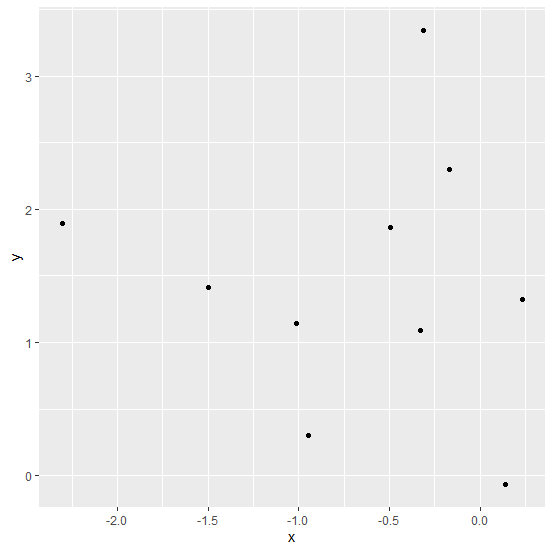# How to extract data from a plot created by ggplot2 in R?

Of course, a plot is created with some data but we might want to get the data from plot as well. This is possible in R with ggplot_build function but it works only for ggplot objects, if we create a plot with plot function then we cannot extract the data with the plot using ggplot_build. Also, it is not necessary that we create the plot using ggplot2 and save it as an object in R to get the data from ggplot_build, we can simply use this function while creating the plot.

## Example

Consider the below data frame −

set.seed(111)
x <-rnorm(10)
y <-rnorm(10,1.5)
df <-data.frame(x,y)
df

## Output

x y
1 0.2352207 1.32632587
2 -0.3307359 1.09340122
3 -0.3116238 3.34563626
4 -2.3023457 1.89405411
5 -0.1708760 2.29752850
6 0.1402782 -0.06666536
7 -1.4974267 1.41414899
8 -1.0101884 1.14086052
9 -0.9484756 0.30639103
10 -0.4939622 1.86418674

Creating the plot with ggplot2 −

## Example

library(ggplot2)
scatterplot <-ggplot(df,aes(x,y))+geom_point()
scatterplot

## OutputExtracting data from the plot −

## Example

data_from_scatterplot <-ggplot_build(scatterplot)
data_from_scatterplot
$data$data[]

## Output

x y PANEL group shape colour size fill alpha stroke
1 0.2352207 1.32632587 1 -1 19 black 1.5 NA NA 0.5
2 -0.3307359 1.09340122 1 -1 19 black 1.5 NA NA 0.5
3 -0.3116238 3.34563626 1 -1 19 black 1.5 NA NA 0.5
4 -2.3023457 1.89405411 1 -1 19 black 1.5 NA NA 0.5
5 -0.1708760 2.29752850 1 -1 19 black 1.5 NA NA 0.5
6 0.1402782 -0.06666536 1 -1 19 black 1.5 NA NA 0.5
7 -1.4974267 1.41414899 1 -1 19 black 1.5 NA NA 0.5
8 -1.0101884 1.14086052 1 -1 19 black 1.5 NA NA 0.5
9 -0.9484756 0.30639103 1 -1 19 black 1.5 NA NA 0.5
10 -0.4939622 1.86418674 1 -1 19 black 1.5 NA NA 0.5

## Example

$layout <ggproto object: Class Layout, gg> coord: <ggproto object: Class CoordCartesian, Coord, gg> aspect: function backtransform_range: function clip: on default: TRUE distance: function expand: TRUE is_free: function is_linear: function labels: function limits: list modify_scales: function range: function render_axis_h: function render_axis_v: function render_bg: function render_fg: function setup_data: function setup_layout: function setup_panel_params: function setup_params: function transform: function super: <ggproto object: Class CoordCartesian, Coord, gg> coord_params: list facet: <ggproto object: Class FacetNull, Facet, gg> compute_layout: function draw_back: function draw_front: function draw_labels: function draw_panels: function finish_data: function init_scales: function map_data: function params: list setup_data: function setup_params: function shrink: TRUE train_scales: function vars: function super: <ggproto object: Class FacetNull, Facet, gg> facet_params: list finish_data: function get_scales: function layout: data.frame map_position: function panel_params: list panel_scales_x: list panel_scales_y: list render: function render_labels: function reset_scales: function setup: function setup_panel_params: function train_position: function xlabel: function ylabel: function super: <ggproto object: Class Layout, gg>$plot
attr(,"class")
 "ggplot_built"